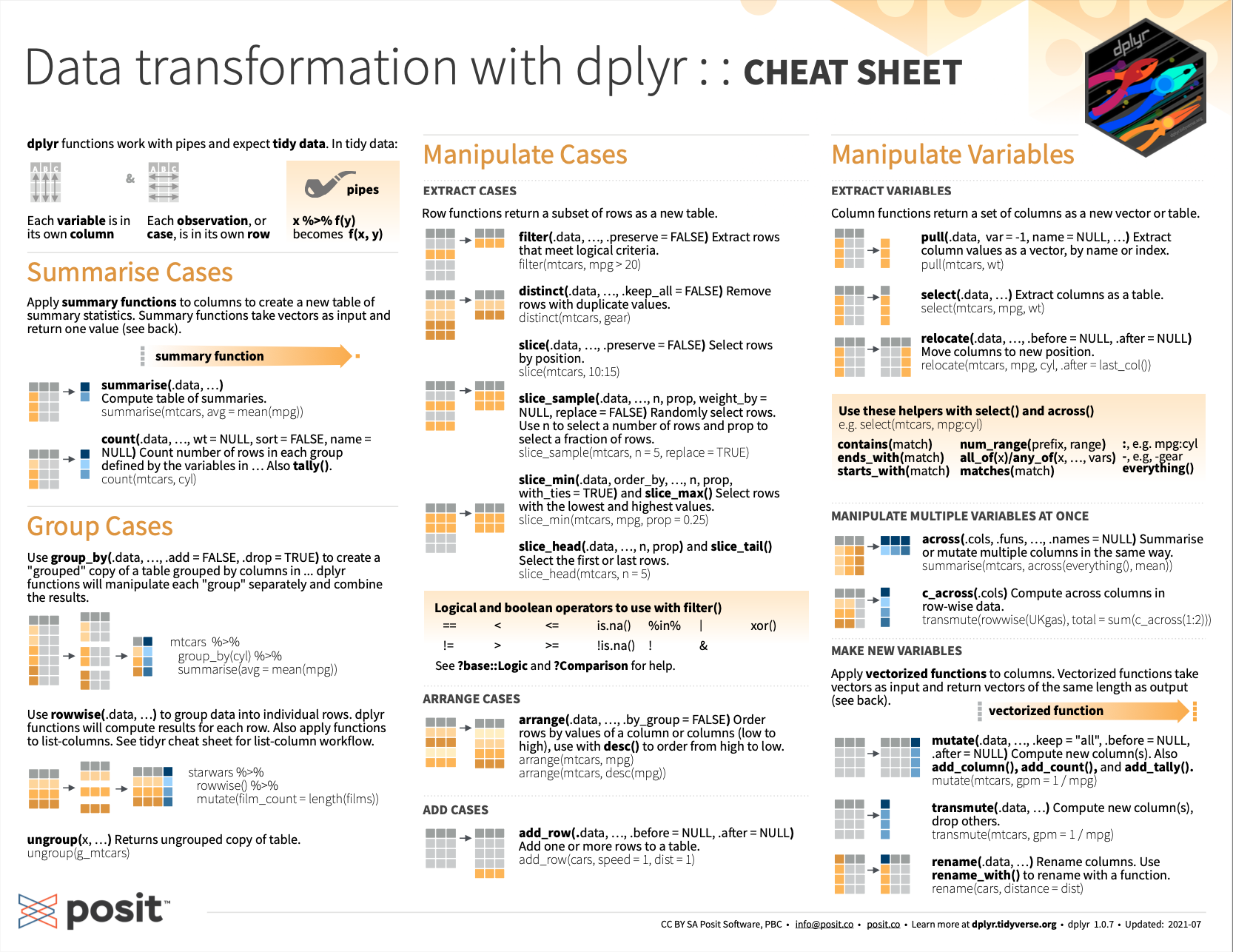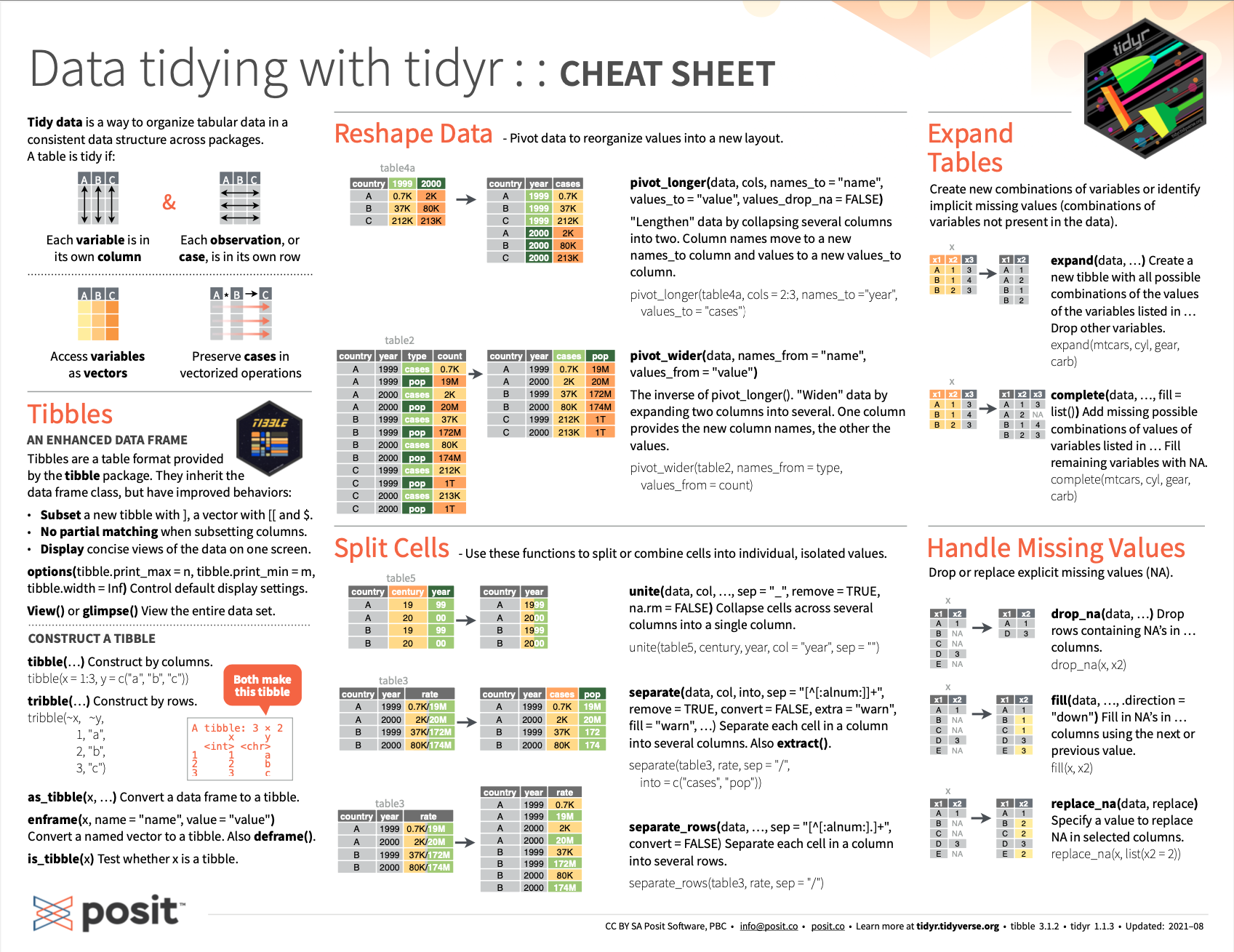## 6.5 Conclusion

### 6.5.1 Summary

This chapter introduced the pipe operator of magrittr and a range of functions for manipulating tables from the tidyverse packages dplyr and tidyr .

So what is the difference between dplyr and tidyr? If we view the functions of both packages as tools, the boundary between both packages is pretty arbitrary. Both packages provide functions for manipulating tables of data. In terms of tasks, the dplyr functions mainly aim to explicate and summarize the data contained in a table, whereas the tidyr functions aim to shape or reshape the table. However, dplyr also provides tools for joining multiple tables and tidyr can be used to select, separate, or unite variables of a table. Thus, the functionalities of both packages are similar enough to think of them as elements of a large toolbox for manipulating data tables — which is why they were covered in a single chapter here.

### 6.5.2 Resources

#### On dplyrFigure 6.3: Data transformation with dplyr from RStudio cheatsheets.

#### On tidyr

• See the cheatsheet on transforming data with tidyr functions (on the back of the Data Import cheatsheet on the readr package) from RStudio cheatsheets:Figure 6.4: The RStudio cheatsheet on reshaping data with tidyr functions (on the back of the Data Import cheatsheet on the readr package).

• For background information on the notion of tidy data, see the following paper by Hadley Wickham (2014b):

### 6.5.3 Preview

We now have all ingredients in place for conducting an exploratory data analysis (EDA). Thus, Chapter 7) will be on exploring data.

### References

Bache, S. M., & Wickham, H. (2014). magrittr: A forward-pipe operator for R. https://CRAN.R-project.org/package=magrittr
Wickham, H. (2014b). Tidy data. Journal of Statistical Software, 59(10), 1–23. https://doi.org/10.18637/jss.v059.i10
Wickham, H., François, R., Henry, L., & Müller, K. (2021). dplyr: A grammar of data manipulation. https://CRAN.R-project.org/package=dplyr
Wickham, H., & Henry, L. (2020). tidyr: Tidy messy data. https://CRAN.R-project.org/package=tidyr